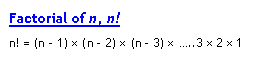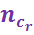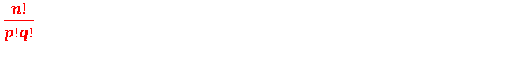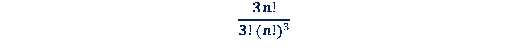Combination Formulas

Factorial of n,n! :The Combinations formula:Important formulas on Combinations:

1. nc0 = 1

2. ncn = 1

3. ncr = ncn-r

4. The number of groups (combinations) of n different things taking any number at a time is:
nc0 + nc1 + nc2 +â€¦.. ncn = 2n â€“ 1
5. The number of ways (alternatively groups or combinations) in which n things can be divided into p things and q things is6. The number of ways (alternatively groups or combinations) in which 3n can be divided equally into three distinct groups each having n things is7. The number of ways (alternatively groups or combinations) in which 3n can be divided equally into three identical groups each having n things is